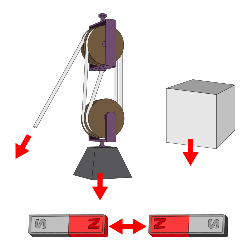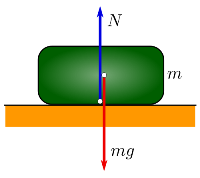Parents and Teachers: Support Ducksters by following us onor.

Science >> Physics for Kids

# Physics for Kids

## Force

What is force?

In physics, force is a push or pull on an object. A force can cause an object to accelerate, slow down, remain in place, or change shape.

How to Measure Force

The unit of measure for force is the newton which is abbreviated as "N". One newton is the force needed to accelerate one gram of mass by one centimeter per second squared. Other units of force include the dyne and the pound-force.Examples of force

Force, Mass, and Acceleration

Force can be figured out if you know the mass and acceleration of an object. This equation comes from Newton's Second Law of Motion:

f = m * a

Where f = force, m = mass, and a = acceleration.

Forces and Vectors

Force not only has a magnitude (which is what we get in newtons when we use the equation above), but it also has a direction. This makes force a vector. Vectors are shown by an arrow that indicates the direction of the force and a number that indicates the magnitude. See the pictures to the right to see how the arrow is used to show the direction of the force.

Forces in Equilibrium

Sometimes there can be many forces acting on an object, but the object remains still. In this case the forces are in equilibrium. The sum of the forces, or the net force, is zero.

The picture below shows an object sitting on a table. The object isn't moving. This is because the force of gravity pulling the object down is equal and opposite to the force of the table pushing up. The net force is zero and the forces are in equilibrium.Combined Forces

When multiple forces are acting on an object, the resultant force is the sum of the vectors of the individual forces. We won't get into complex vector math here, but take for example a tug of war. The two sides are each pulling. If one side is pulling with a force of 2 N in left direction and the other side is pulling with a force of 3 N in the right direction, then the resultant force is 1 N in the right direction.

Types of Forces
• Friction - Friction is a force caused when one object rubs against another. It works in the opposite direction of the main force.
• Gravity - Gravity is a force caused by a large body, such as the Earth. Gravity pulls objects toward the Earth with an acceleration of "g" which equals 9.8 m/s2.
• Electromagnetic - Electromagnetic force is a force associated with electric and magnetic fields.
• Nuclear - Nuclear forces are the forces that hold atoms and their particles together.
• Tension - A pulling force that is exerted by a string, cable, or chain on another object.
• Elastic - An elastic force is a force exerted by an object trying to return to its natural length. This is modeled by a spring that has been pulled by an external force, but is pulling back while trying to return to its original length.
Interesting Facts about Force
• An object that is accelerating in a circular motion experiences "centripetal" force.
• The four fundamental forces are gravity, electromagnetic force, the strong nuclear force, and the weak nuclear force.
• Torque is a type of force that measures changes in the rotational speed of an object. Torque is an important feature of automobiles, especially trucks.
• Drag is a force that decreases the velocity of an object. Thrust is a force that increases the velocity of an object.
Activities

More Physics Subjects on Motion, Work, and Energy

Science >> Physics for KidsFollow us onor